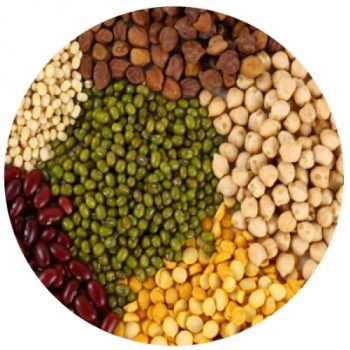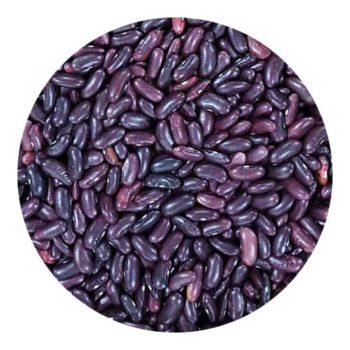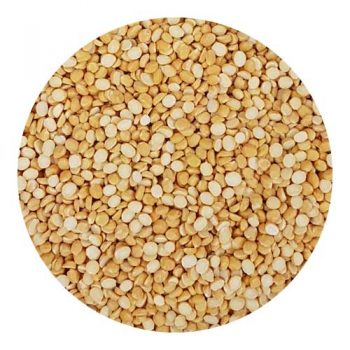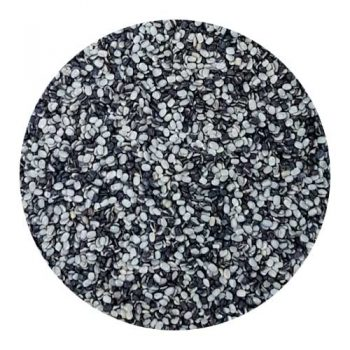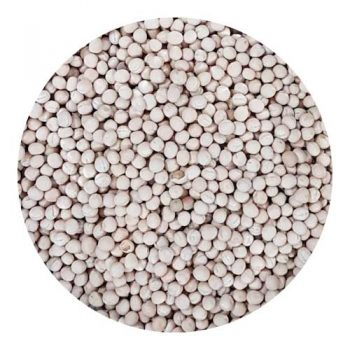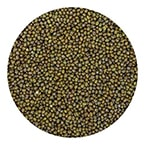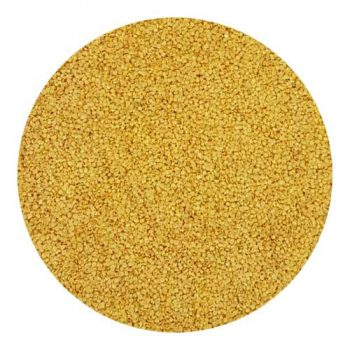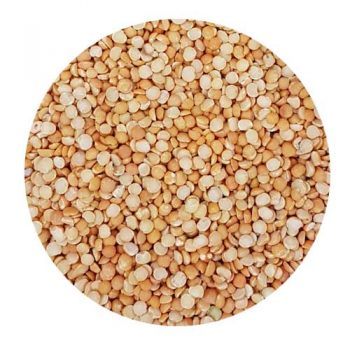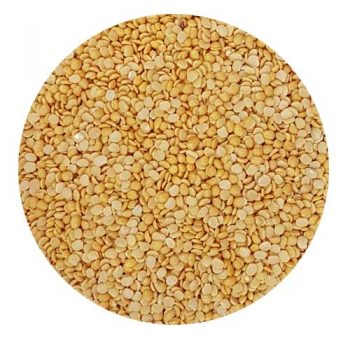## Dals & Pulses

27 Incl. Tax

Quick Order From our Dal and Pulses Menu, you can Blindly trust our quality. Soon you will realise why we say Accha Khao, Maze se Raho – Since 70 Years…..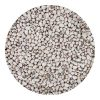Barbati Weight Choose Weight250gm - Rs. 27500gm - Rs. 501kg - Rs. 962kg - Rs. 1881.5kg - Rs. 1422.5kg - Rs. 2343kg - Rs. 280 Clear ₹27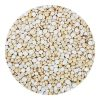Batana Daal Weight Choose Weight1kg - Rs. 922kg - Rs. 180500gm - Rs. 481.5kg - Rs. 1362.5kg - Rs. 2243kg - Rs. 268 Clear ₹48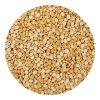Chana Daal Weight Choose Weight500gm - Rs. 501kg - Rs. 962kg - Rs. 1881.5kg - Rs. 1422.5kg - Rs. 2343kg - Rs. 280 Clear ₹50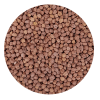Desi Chana Weight Choose Weight500gm - Rs. 461kg - Rs. 882kg - Rs. 1721.5kg - Rs. 1302.5kg - Rs. 2143kg - Rs. 256 Clear ₹46Daliya Weight Choose Weight250gm - Rs. 34500gm - Rs. 631kg - Rs. 1211.5kg - Rs. 1792.5kg - Rs. 2953kg - Rs. 353 Clear ₹34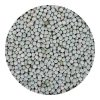Hara Batana Weight Choose Weight500gm - Rs. 571kg - Rs. 1101.5kg - Rs. 1632.5kg - Rs. 2693kg - Rs. 322 Clear ₹57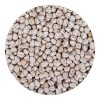Kabuli Chana Weight Choose Weight500gm - Rs. 991kg - Rs. 1942kg - Rs. 3841.5kg - Rs. 2892.5kg - Rs. 4793kg - Rs. 574 Clear ₹99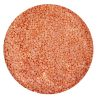Masoor Daal Weight Choose Weight200gm - Rs. 22500gm - Rs. 481kg - Rs. 922kg - Rs. 1801.5kg - Rs. 1362.5kg - Rs. 2243kg - Rs. 268 Clear ₹22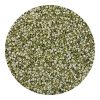Moong Daal Chilka Weight Choose Weight500gm - Rs. 661kg - Rs. 1282kg - Rs. 2521.5kg - Rs. 1902.5kg - Rs. 3143kg - Rs. 376 Clear ₹66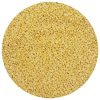Moong Mogar Weight Choose Weight500gm - Rs. 691kg - Rs. 1342kg - Rs. 2641.5kg - Rs. 1992.5kg - Rs. 3293kg - Rs. 394 Clear ₹69Moong Sabut Weight Choose Weight500gm - Rs. 671kg - Rs. 1302kg - Rs. 2561.5kg - Rs. 1932.5kg - Rs. 3193kg - Rs. 382 Clear ₹67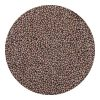Motha Weight Choose Weight500gm - Rs. 621kg - Rs. 1202kg - Rs. 2361.5kg - Rs. 1782.5kg - Rs. 2943kg - Rs. 352 Clear ₹62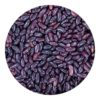Rajma Weight Choose Weight250gm - Rs. 47500gm - Rs. 891kg - Rs. 1741.5kg - Rs. 2592kg - Rs. 3443kg - Rs. 514 Clear ₹47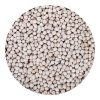Safed Batana Weight Choose Weight250gm - Rs. 24500gm - Rs. 441kg - Rs. 841.5kg - Rs. 1242.5kg - Rs. 2043kg - Rs. 244 Clear ₹24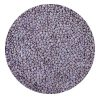Masoor Saiga Whole Weight Choose Weight250gm - Rs. 26500gm - Rs. 481kg - Rs. 921.5kg - Rs. 1362.5kg - Rs. 2243kg - Rs. 268 Clear ₹26Urad Saiga Whole Weight Choose Weight250gm - Rs. 34500gm - Rs. 641kg - Rs. 1241.5kg - Rs. 1842.5kg - Rs. 3043kg - Rs. 364 Clear ₹34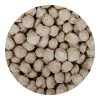Soyabean Badi Weight Choose Weight100gm - Rs. 14200gm - Rs. 24250gm - Rs. 29300gm - Rs. 34500gm - Rs. 54 Clear ₹14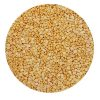Tur Daal Supreme Weight Choose Weight500gm - Rs. 991kg - Rs. 1942kg - Rs. 3841.5kg - Rs. 2892.5kg - Rs. 4793kg - Rs. 574 Clear ₹99Urad Daal Chilka Weight Choose Weight250gm - Rs. 36500gm - Rs. 671kg - Rs. 1301.5kg - Rs. 1932.5kg - Rs. 3193kg - Rs. 382 Clear ₹36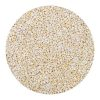Urad Daal Mogar Weight Choose Weight250gm - Rs. 37500gm - Rs. 691kg - Rs. 1342kg - Rs. 2641.5kg - Rs. 1992.5kg - Rs. 329 Clear ₹37